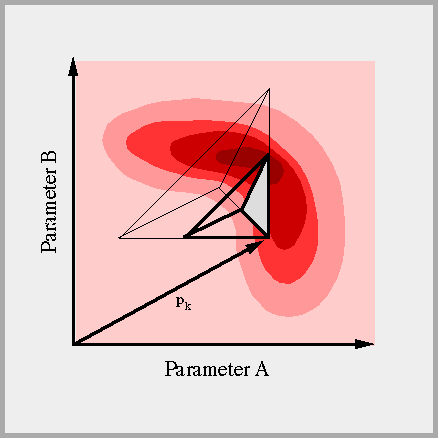# Minimization Algorithm

#### Minimization AlgorithmsThe purpose of the minimization algorithm is to detect the minimum of the objective function in the n-dimensional parameter space. In multiphase flow modeling, the calculated system response is a highly non-linear function of the input parameters. Consequently, the objective function is non-quadratic when applying least-squares. Starting from an initial parameter set, the minimum has to be found iteratively by evaluating the value and/or gradient of the objective function, and performing small steps towards the minimum. Different minimization algorithms are available in iTOUGH2 , each with its advantages and disadvantages. The choice depends on the characteristics of the objective function, the number of parameters to be estimated, and the efficiency with which the forward problem can be solved.#### Levenberg-Marquardt AlgorithmThe Levenberg-Marquardt modification of the Gauss-Newton algorithm for the minimization of non-quadratic objective functions was found to be an efficient and robust method for most iTOUGH2 inversions. After local linearization of the objective function with respect to the parameters to be estimated, the Levenberg-Marquardt algorithm performs initially small, but robust steps along the steepest descent direction, and switches to more efficient quadratic Gauss-Newton steps as the minimum is approached. The derivatives are calculated numerically using the perturbation method.#### Downhill Simplex AlgorithmThe downhill simplex method requires only function evaluations (i.e., no derivatives) and is therefore a robust but inefficient minimization method. Starting with a simplex consisting of n+1 points in the n-dimensional parameter space, a series of steps is taken, most of which just moving the point of the simplex with the highest objective function through the opposite face of the simplex to a lower point. Other search directions are generated by reflection, expansion, and contraction of the simplex from the previous step. The simplex algorithm should be used in iTOUGH2 when minimizing discontinuous cost functions or if the numerical evaluation of the derivatives is unstable.#### Simulated AnnealingA continuous version of the method of simulated annealing has been implemented in iTOUGH2. Simulated annealing is a technique to find the (ideally global) minimum of the objective function in the presence of many local minima. Random steps in the parameter space are performed. A step is always accepted if the objective function is lowered, and it is sometimes accepted with a certain, decreasing probability, if an uphill step is taken. This scheme allows the algorithm of escaping from local minima. Simulate annealing requires many forward runs and is thus only applicable to small problems.The global minimum can always be identified by evaluating the objective function in the entire parameter space. Moreover, contouring the objective function reveals the potential presence of local minima, non-uniqueness problems, the correlation structure, stability problems, and non-linearity effects. Evaluating the objective function on a grid in the entire parameter space is prohibitively expensive for higher dimensional parameter spaces. iTOUGH2 offers this option for up to three parameters. This example of a two-dimensional grid search shows the objective function in the parameter space spanned by the logarithm of the absolute permeability and the pore size distribution index of the Brooks-Corey model. A three-dimensional grid search has been performed to generate the picture shown at the top of this page.